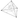Dissection into Orthoschemes, the Hadwiger Conjecture

The following (unsolved in the general case) conjecture is due to Hadwiger:

(Hadwiger’s Conjecture, 1956) In Euclidean space, in any dimension, any simplex can always be dissected into orthoschemes.

Orthoschemes (or Schläfli orthoschemes, or path-simplices in the CS/applied math literature), are a generalisation of right-angled triangles in the plane. You can think of them as a sequence of $d$ edges all pairwise orthogonal where $d$ is the dimension (e.g the two orthogonal edges of a right-angled triangle in the plane, the three mutually orthogonal edges of a birectangular tetrahedron in space).

The conjecture has been validated in dimension up to 5:

(In Dimension 2) Any simplex can be dissected in 2 orthoschemes (minimal)
(In Dimension 3) Any simplex can be dissected in 12 orthoschemes (minimal)
(In Dimension 4) Any simplex can be dissected in 500 orthoschemes
(In Dimension 5) Any simplex can be dissected in 12.5 million orthoschemes

Over the summer I was working on acute triangulations of polyhedra and my first task was to verify that every simplex can be dissected into non-obtuse simplices. I later found out that this was a known conjecture (funny how the internet keeps you in the dark if you don’t know the linguo in the math community for this, the word orthoscheme in my case) and had been proved up to dimension 5. Since we originally had publication in mind, I typed up the proof nicely in this pdf. On the plus side my proof is different and more rigorous than the original one published in some obscure German paper, but overall it’s essentially the same.

You can retrieve his minimal dissection (12 tetrahedra are sufficient, whereas my construction uses 28) by paying individual attention to the 6 cases in Figure 4 (only II, III and IV are interesting) and it should be easy to prove minimality using Euler characteristic combinatorics and minimal non-obtuse triangulations of the spherical links of the vertices in original tetrahedron.

My first working proof relied on spherical geometry and spherical triangles in the links, but I later realised this was unnecessary. But as an added bonus this allowed me to classify minimal non-obtuse dissections of spherical triangles - one needs either 2, 4 or 6 depending on the angles/lengths of the triangle.

Naively, I thought an induction on the dimension would yield the proof in the general case, assuming we can proove non-existence of acute cycles in higher dimensions we should be able to project the centre of the inscribed sphere on all faces and use a dissection of these faces (by induction) to build the new dissection. But it’s definitely not clear how to go from that $n-1$ dimensional dissection to the $n$-dimensional one, even though we project the point perpendicularly on the face, even in the 3 dimensional case, we’re not just using non-existence of acute cycles to prove we get a valid dissection but we’re using a very specific construction. After spending some thoughts, it’s definitely not trivial to generalise that construction even to the 4 dimensional case.